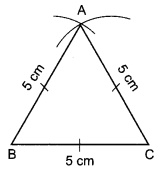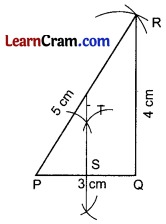# DAV Class 7 Maths Chapter 10 Worksheet 1 Solutions

The DAV Class 7 Maths Solution and DAV Class 7 Maths Chapter 10 Worksheet 1 Solutions of Construction of Triangles offer comprehensive answers to textbook questions.

## DAV Class 7 Maths Ch 10 WS 1 Solutions

Question 1.
Construct a ΔLMN in which LM = 5 cm, MN = 5.6 cm and NL = 4.2 cm.
Steps of Construction:1. Draw MN = 5.6 cm.
2. Take M and N as centre and draw two arcs with radius 5 cm and 4.2 cm respectively.
3. L is the point of intersection of the two arcs.
4. Join ML and NL.
5. ALMN is the required triangle.

Question 2.
Draw ΔDEF such that DE = DF = 4 cm and EF = 6 cm.
Steps of Construction:1. Draw EF = 6cm.
2. Draw two arcs with centre E and F and radius 4 cm.
3. Let the two arcs be cut at D.
4. Join ED and FD.
5. ΔDEF is the required triangle.Question 3.
Draw an equilateral triangle one of whose sides is of length 5 cm.
Steps of Construction:1. Draw BC = 5 cm.
2. Draw two arcs with centre B and C and with radius 5 cm.
3. Let the two arcs be cut at A.
4. Join AB and AC.
5. ΔABC is the required equilateral triangle.

Question 4.
Draw A PQR in which QR = 4 cm, PQ = 3 cm, RP = 5 cm. Also draw perpendicular bisector of side PQ.
Steps of Construction:1. Draw PQ = 3 cm.
2. Draw the arcs with centre P and Q and with radius 5 cm and 4 cm respectively.
3. Let the two arcs be cut at R.
4. Join PR and QR.
5. ΔPQR is the required triangle.
6. Draw a perpendicular bisector ST at PQ.Question 5:
Is it possible to construct a triangle whose sides are 3 cm, 6.1 cm and 2.6 cm?
To construct a triangle, the necessary condition is that the sum of any two sides of the triangle must be greater than the third side.
Here, 3 cm + 2.6 cm < 6.1 cm
⇒ 5.6 cm < 6.1 cm
Hence, triangle is not possible to construct.

Question 6.
Draw a ΔPQR in which PQ = 7 cm, QR = 3 cm, RP = 4 cm. Is the construction of triangle possible?
Here, PQ = 7 cm, QR = 3cm and RP = 4 cm
QR + RP = PQ
3 + 4 = 7
7 = 7
∴ P, Q and R are collinear
Hence, the triangle is not possible to construct.### DAV Class 8 Maths Chapter 10 Worksheet 1 Notes

If A, B, C are three non-collinear points, the figure made by three line segments AB, BC and CA is called the triangle with vertex A, B, C.
The three line segments forming a triangle are called the sides of the triangle.

While constructing a triangle, the following facts are to be kept in mind.

• The sum of the three angles of a triangle is 180°.
• The sum of any two sides of a triangle is greater than the third side.
• There are six components of a triangle, three angles and three sides.
• Triangle is possible to construct if any three components are known independently.

A triangle can be constructed when

•  All the three sides are given. (SSS triangle construction)
• Two sides and an included angle are given. (SAS triangle construction)
• Two angles and an included side are given. (ASA triangle construction)
• Right angle with hypotenuse and one side are given. (RHS triangle construction)Example 1:
Construct a Δ PQR in which PQ = 3cm, PR = 4 cm and QR = 5 cm. What type of this triangle is?
Solution:
Steps of construction:

1. Draw QR = 5 cm.
2. Draw two arcs with radius 3 cm and 4 cm taking centres Q and R respectively to meet each other at P.
3. Join PQ and PR.
4. Δ PQR is the required triangle.
5. Δ PQR is a right angled triangle.Example 2:
Construct a triangle whose any two sides are 3.5 cm and 4.5 cm and the included angle is 60°.
Solution:
Steps of Construction:

1. Draw BC = 3.5 cm
2. Draw ∠B = 600
3. Cut BA = 4.5 cm B
4. Join AC
5. Δ ABC is the required triangle.Example 3:
Construct Δ ABC in which ∠B = 60°, ∠C = 40° and BC = 4 cm.
Solution:
Steps of Construction:

1. Draw BC = 4 cm.
2. Draw ∠B = 60° and ∠C = 40° to meet each other at A.
3. Δ ABC is the required triangle.Example 4:
Draw a right angle triangle whose hypotenuse is 5.5 cm and a side is 3.5 cm.